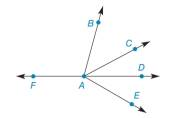Chapter 1.4, Problem 10E### Elementary Geometry for College St...

6th Edition
Daniel C. Alexander + 1 other
ISBN: 9781285195698

#### Solutions

Chapter
Section### Elementary Geometry for College St...

6th Edition
Daniel C. Alexander + 1 other
ISBN: 9781285195698
Textbook Problem
15 views

# Suppose that A B ⇀ , A C ⇀ , A D ⇀ , A E ⇀ , and A F ⇀ are coplanar,Exercises 1 0 - 1 3 Classify the following as true or false:a) m ∠ B A C + m ∠ C A D = m ∠ B A D b) ∠ B A C ≅ ∠ C A D c) m ∠ B A E − m ∠ D A E = m ∠ B A C d) ∠ B A C  and ∠ D A E are adjacente) m ∠ B A C + m ∠ C A D + m ∠ D A E = m ∠ B A E

To determine

a)

To check:

The given statement is true or false.

Explanation

If a point say M lies in the interior of PQR, then mPQM+mRQM=mPQR.

Given:

The given statement is,

The given figure is,

Approach:

The given statement is,

To determine

b)

To check:

The given statement is true or false.

To determine

c)

To check:

The given statement is true or false.

To determine

d)

To check:

The given statement is true or false.

To determine

e)

To check:

The given statement is true or false.

### Still sussing out bartleby?

Check out a sample textbook solution.

See a sample solution

#### The Solution to Your Study Problems

Bartleby provides explanations to thousands of textbook problems written by our experts, many with advanced degrees!

Get Started